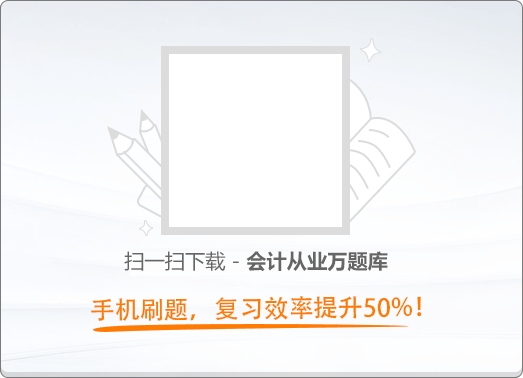60:00《财经法规与会计职业道德》2016新大纲真题精编（二）

1
(单项选择题)

《中华人民共和国会计法》规定单位内部会计监督的主体是（　）。

• A.

本单位的会计机构和会计人员

• B.

上级主管部门

• C.

本单位的主要领导

• D.

本单位的监察部门

• A
• B
• C
• D

2
(单项选择题)

• A.

电话号码

• B.

身份证

• C.

支票存根

• D.

预留银行印章

• A
• B
• C
• D

3
(单项选择题)

• A.

指导功能

• B.

评价功能

• C.

教化功能

• D.

法治功能

• A
• B
• C
• D

4
(单项选择题)

• A.

会计年度终了后第一天

• B.

每月终了后第一天

• C.

经济业务发生日

• D.

会计档案装订日

• A
• B
• C
• D

5
(单项选择题)

• A.

可由移交人员自行授权他人代办移交

• B.

经单位负责人批准，可由移交人员委托他人代办移交

• C.

经会计机构负责人批准，可由移交人员委托他人代办移交

• D.

可由会计机构负责人自行授权他人代办移交

• A
• B
• C
• D

6
(单项选择题)

• A.

私设会计账簿

• B.

变造会计账簿

• C.

随意变更会计处理方式

• D.

伪造会计账簿

• A
• B
• C
• D

7
(单项选择题)

• A.

指令其参加一定学时的继续教育课程

• B.

行业范围内通报批评

• C.

吊销会计从业资格

• D.

在行业内部公共刊物上曝光

• A
• B
• C
• D

8
(单项选择题)

• A.

身体健康

• B.

从事会计工作重要工作岗位满2年

• C.

坚持原则，廉洁奉公

• D.

取得会计师任职资格后，主管一个单位的财务会计工作时间满3年

• A
• B
• C
• D

9
(单项选择题)

• A.

出票日期

• B.

收款人名称

• C.

票据金额

• D.

用途

• A
• B
• C
• D

10
(单项选择题)

• A.

用现金结算银行存款利息

• B.

用现金结算贷款

• C.

用信用卡结算贷款

• D.

用现金结算银行贷款利息

• A
• B
• C
• D

11
(单项选择题)

• A.

从事货物生产兼营批发的纳税人，年应税销售额在50万元下的

• B.

从事货物批发的纳税人，年应税销售额在100万元以下的

• C.

从事货物生产兼营批发的纳税人，年应税销售额在80万元以下的

• D.

从事货物零售的纳税人，年应税销售额在100万元以下的

• A
• B
• C
• D

12
(单项选择题)

• A.

2

• B.

3

• C.

5

• D.

10

• A
• B
• C
• D

13
(单项选择题)

• A.

省级以上人民政府财政部门

• B.

县级以上人民政府财政部门

• C.

工商行政管理部门

• D.

商务部

• A
• B
• C
• D

14
(单项选择题)

• A.

生产应税消费品的单位

• B.

进口应税消费品的单位

• C.

委托加工应税消费品的个人

• D.

受托加工应税消费品的单位

• A
• B
• C
• D

15
(单项选择题)

• A.

具有特殊性，只能从有限范围的供应商处采购的

• B.

招标后没有供应商投标或者没有合格标的或者重新招标未能成立的

• C.

采用公开招标方式的费用占政府采购项目总价值的比例过小的

• D.

采用招标所需时间不能满足用户紧急需要的

• A
• B
• C
• D

16
(单项选择题)

• A.

取得会计从业资格证书

• B.

具有会计师以上专业资格

• C.

从事会计工作3年以上

• D.

有主管会计工作的经历

• A
• B
• C
• D

17
(单项选择题)

• A.

3 000元以上20 000元以下

• B.

5 000元以上30 000元以下

• C.

10 000元以上30 000元以下

• D.

5 000元以上10 000元以下

• A
• B
• C
• D

18
(单项选择题)

• A.

设立临时机构

• B.

异地临时经营活动

• C.

注册验资

• D.

短期借款

• A
• B
• C
• D

19
(单项选择题)

• A.

出纳

• B.

稽核

• C.

会计机构负责人

• D.

药房收费员

• A
• B
• C
• D

20
(单项选择题)

• A.

10

• B.

30

• C.

60

• D.

90

• A
• B
• C
• D